# Proof of Sine double angle identity

The sine of double angle identity is a trigonometric identity and used as a formula. It is usually written in the following three popular forms for expanding sine double angle functions in terms of sine and cosine of angles.

$(1).\,\,\,$ $\sin{(2\theta)}$ $\,=\,$ $2\sin{\theta}\cos{\theta}$

$(2).\,\,\,$ $\sin{(2A)}$ $\,=\,$ $2\sin{A}\cos{A}$

$(3).\,\,\,$ $\sin{(2x)}$ $\,=\,$ $2\sin{x}\cos{x}$

The sine double angle rule can be proved in mathematical form geometrically from a right triangle (or right angled triangle). It is your turn to learn the geometric proof of sin double angle formula.

## Construction

Let’s consider a right angled triangle. Here, it is $\Delta ECD$. We have to do some geometric settings inside this right triangle by following below steps.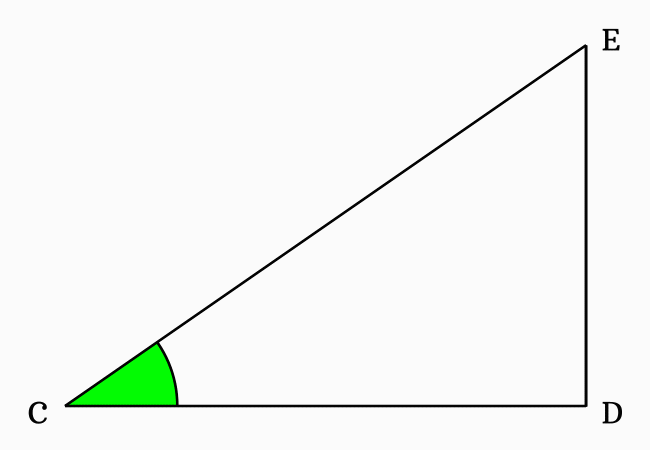1. Bisect the angle $DCE$ by drawing a straight line from point $C$ to side $\overline{DE}$ and it intersects the side $\overline{DE}$ at point $F$. Each angle is represented by a variable $x$.
2. Draw a line to side $\overline{CE}$ from point $F$ but it should be perpendicular to side $\overline{CF}$. Assume that it intersects the side $\overline{CE}$ at point $G$.
3. Draw a perpendicular line to side $\overline{CD}$ from point $\small G$. It intersects the side $\overline{CF}$ at point $H$ and perpendicularly intersects side $\overline{CD}$ at point $I$.
4. Draw a perpendicular line to side $\small \overline{GI}$ from point $\small F$ and it intersects the side $\small \overline{GI}$ at point $\small J$.

Now, we can start deriving the expansion of the sine of double angle trigonometric identity in mathematical form.

### Procedure

When $x$ is used to represent an angle of a right triangle, the sine, cosine and sine of double angle functions are written as $\sin{x}$, $\cos{x}$ and $\sin{2x}$ respectively.

### Express the Sine of double angle in Ratio form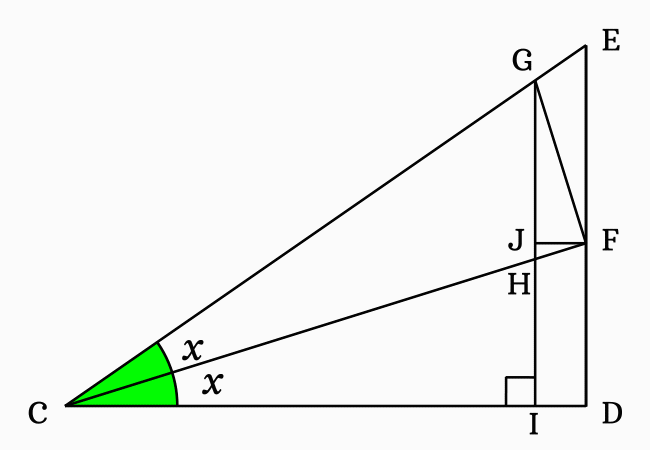The side $\overline{CF}$ bisects the $\angle DCE$ as two equal angles $\angle DCF$ and $\angle FCE$. Here, the $\angle DCF$ and $\angle FCE$ are equal and each angle is denoted by $x$.

$\angle DCE$ $\,=\,$ $\angle DCF + \angle FCE$

$\implies$ $\angle DCE \,=\, x+x$

$\,\,\,\therefore\,\,\,\,\,\,$ $\angle DCE \,=\, 2x$

It is cleared that the angle $DCE$ is equal to $2x$. Hence, the sine of angle $2x$ is written as $\sin{(2x)}$ in trigonometric mathematics.

According to the $\Delta ICG$, the sine of double angle can be written in ratio form of the lengths of the sides.

$\sin{2x} \,=\, \dfrac{GI}{CG}$

The side $\overline{JF}$ splits the side $\overline{GI}$ as two sides $\overline{GJ}$ and $\overline{JI}$. So, the length of side $\overline{GI}$ can be written as the sum of them mathematically.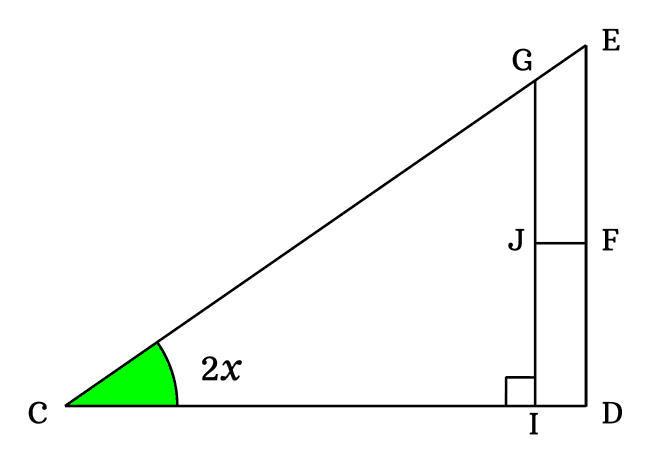$GI = GJ + JI$

Now, replace the length of side $\overline{GI}$ by its equivalent value in the expansion of $\sin{(2x)}$ function.

$\implies$ $\sin{2x}$ $\,=\,$ $\dfrac{GJ+JI}{CG}$

$\implies$ $\sin{2x}$ $\,=\,$ $\dfrac{GJ}{CG}+\dfrac{JI}{CG}$

The sides $\overline{GI}$ and $\overline{DE}$ are parallel lines. So, the length of the side $\overline{JI}$ is exactly equal to the length of the side $\overline{DF}$. Therefore, $JI = DF$.

$\implies$ $\sin{2x}$ $\,=\,$ $\dfrac{GJ}{CG}+\dfrac{DF}{CG}$

#### Express trigonometric ratios in functions

The $\sin{(2x)}$ is expanded in terms of the ratios between the sides. Now, let’s try to express each ratio in the form a trigonometric function.

The side $\overline{EF}$ is opposite side of the $\Delta ECF$ and its angle is $x$. Try to write the length of side $\overline{EF}$ in terms of a trigonometric function.$\sin{x} = \dfrac{EF}{CF}$

$\implies EF = {CF}\sin{x}$

Now, substitute the length of perpendicular $\overline{EF}$ by its equivalent value in the expansion of $\sin{2x}$.

$\implies$ $\sin{2x} \,=\, \dfrac{GJ}{CG}+\dfrac{{CF}\sin{x}}{CG}$

$\implies$ $\sin{2x} \,=\, \dfrac{GJ}{CG}+\dfrac{CF}{CG} \times \sin{x}$

The sides $\overline{CF}$ and $\overline{CG}$ are sides of the right triangle $GCF$ and the angle of this triangle is also $x$. According to trigonometry, the ratio of them can be represented by $\cos{x}$.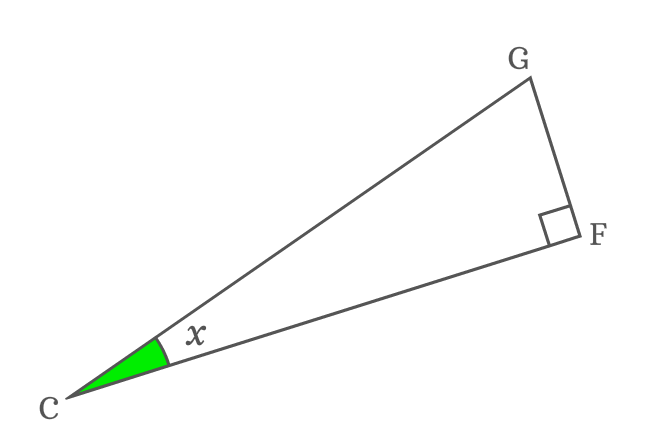$\cos{x} = \dfrac{CF}{CG}$

Now, replace the ratio of lengths of sides $\overline{CF}$ to $\overline{CG}$ by its equivalent trigonometric function in the expansion of sin of double angle function.

$\implies$ $\sin{2x} \,=\, \dfrac{GJ}{CG}+\cos{x} \times \sin{x}$

$\implies$ $\sin{2x} \,=\, \dfrac{GJ}{CG}+\cos{x}\sin{x}$

$\implies$ $\sin{2x} \,=\, \dfrac{GJ}{CG}+\sin{x}\cos{x}$

#### Geometric Analysis for unknown angle

The side $\overline{GJ}$ is one of the sides of the $\Delta JGF$ but its angle is unknown. So, it is not possible to express the length of the side $\overline{GJ}$ in terms of a trigonometric function. Hence, let’s find the angle of this triangle geometrically.

The sides $\overline{CE}$ and $\overline{JF}$ are parallel lines and $\overline{CF}$ is their transversal. $\angle ECF$ and $\angle JFC$ are interior alternate angles and they are equal geometrically.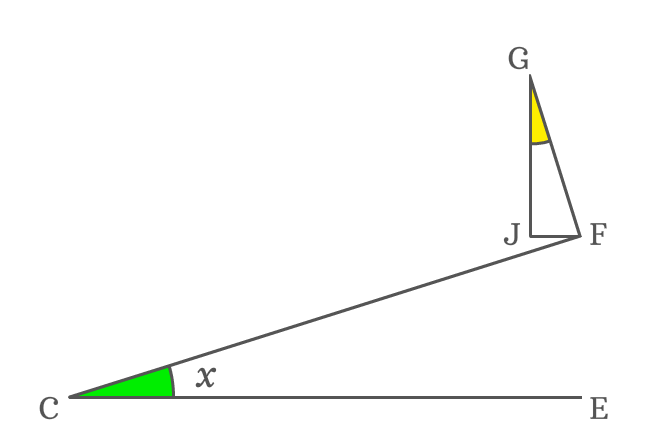$\angle JFC = \angle ECF = x$

The side $\overline{FG}$ is a perpendicular line to side $\overline{CF}$. So, the $\angle CFG = 90^°$. but the side $\overline{JF}$ splits the $\angle CFG$ as $\angle JFC$ and $\angle JFG$.

$\angle JFC + \angle JFG = 90^°$

It is proved about that $\angle JFC = x$.

$\implies x + \angle JFG = 90^°$

$\implies \angle JFG = 90^°-x$

$\Delta JGF$ is a right triangle in which $\angle GJF = 90^°$ and $\angle JFG = 90^°-x$. The $\angle JGF$ can be calculated by the sum of angles rule of a triangle.

$\angle JGF + \angle GJF + \angle JFG$ $=$ $180^°$

$\implies \angle JGF + 90^° + 90^°-x$ $=$ $180^°$

$\implies \angle JGF + 180^°-x$ $=$ $180^°$

$\implies \angle JGF$ $=$ $180^°-180^°+x$

$\,\,\, \therefore \,\,\,\,\,\, \angle JGF = x$

It is proved that the $\angle JGF$ is also equal to $x$ because it is a similar triangle.

#### Write trigonometric ratio in functions

$\sin{2x} \,=\, \dfrac{GJ}{CG}+\sin{x}\cos{x}$

Now, let’s try to write the ratio between sides $\overline{GJ}$ and $\overline{CG}$ in terms of trigonometric functions to get the expansion of sin double angle identity.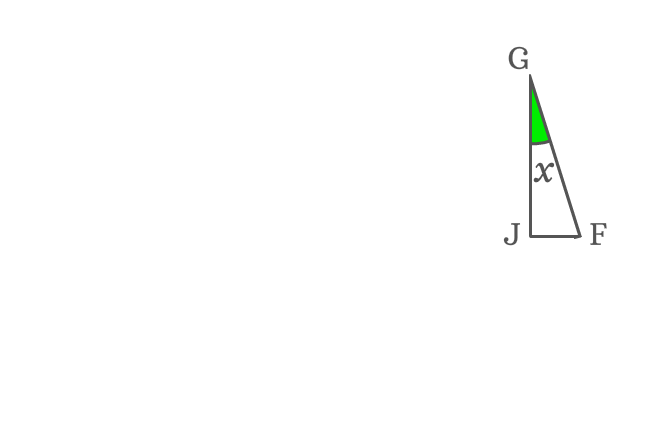$\overline{GJ}$ is adjacent side of $\Delta JGF$ and its angle is derived as $x$.

$\cos{x} = \dfrac{GJ}{GF}$

$\implies GJ = {GF}\cos{x}$

Substitute the length of $\overline{GJ}$ by its equivalent value in the expansion of $\sin{(2x)}$ function.

$\sin{2x}$ $\,=\,$ $\dfrac{{GF}\cos{x}}{CG}+\sin{x}\cos{x}$

$\implies \sin{2x}$ $\,=\,$ $\dfrac{GF}{CG} \times \cos{x}+\sin{x}\cos{x}$

The sides $\overline{GF}$ and $\overline{CG}$ are sides of $\Delta GFC$. So, consider right triangle $GFC$ once more time.$\sin{x} = \dfrac{GF}{CG}$

Therefore, replace the ratio of lengths of sides $\overline{GF}$ to $\overline{CG}$ by trigonometric function $\sin{x}$ in the $\sin{2x}$ expansion.

$\implies \sin{2x}$ $\,=\,$ $\sin{x} \times \cos{x}+\sin{x}\cos{x}$

$\implies \sin{2x}$ $\,=\,$ $\sin{x}\cos{x}+\sin{x}\cos{x}$

$\,\,\, \therefore \,\,\,\,\,\, \sin{2x}$ $\,=\,$ $2\sin{x}\cos{x}$

Therefore, it is proved geometrically that the sin of double angle function can be expanded as two times the product of sin of angle and cos of angle.

Latest Math Topics
Jun 26, 2023
Jun 23, 2023

###### Math Questions

The math problems with solutions to learn how to solve a problem.

Learn solutions

Practice now

###### Math Videos

The math videos tutorials with visual graphics to learn every concept.

Watch now

###### Subscribe us

Get the latest math updates from the Math Doubts by subscribing us.